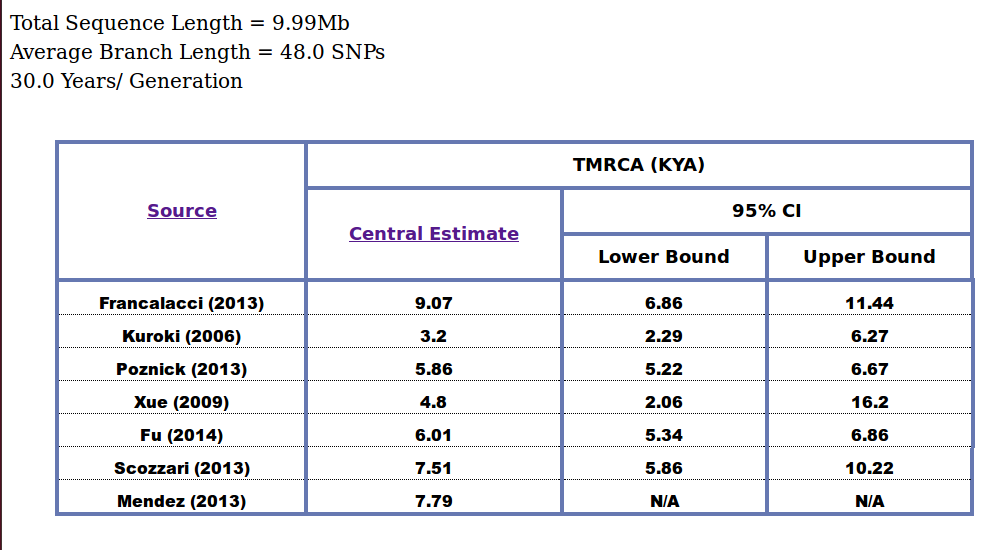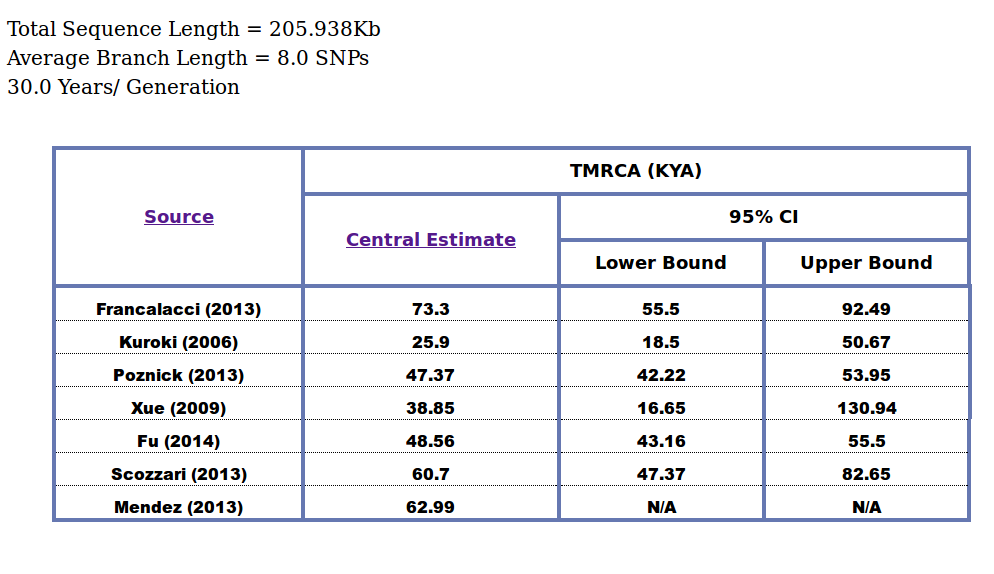## Tuesday, February 3, 2015

### SNP based module added to the Y TMRCA calculator

The solely STR based Y TMRCA calculator now also can accept SNP based input to compute the TMRCA of a node. Instructions and methodology can be found within the app at the link below:
https://ehelix.pythonanywhere.com/init/default/index

For now, it uses 7 separate mutation rates that all come from different publications, but not all necessarily using differing methods to derive the rates. I will look to expand these as more substitution mutation rates become available.

Below I have run some quick verifications for 3 separate mutation rate sources:

Poznick (2013) rates via Underhill (2014)

The following is stated in Underhill (2014):
A consensus has not yet been reached on the rate at which Y-chromosome SNPs accumulate within this 9.99Mb sequence. Recent estimates include one SNP per: ~100 years,⁵⁸ 122 years,⁴ 151 years⁵ (deep sequencing reanalysis rate), and 162 years.⁵⁹ Using a rate of one SNP per 122 years, and based on an average branch length of 206 SNPs from the common ancestor of the 13 sequences, we estimate the bifurcation of R1 into R1a and R1b to have occurred ~25,100 ago (95% CI: 21,300–29,000). Using the 8 R1a lineages, with an average length of 48 SNPs accumulated since the common ancestor, we estimate the splintering of R1a-M417 to have occurred rather recently, B5800 years ago (95% CI: 4800–6800). The slowest mutation rate estimate would inflate these time estimates by one third, and the fastest would deflate them by 17%.
Putting in the variables for the R1 node from above into the calculator,
We get an output of:R1 - Underhill (2014)
which for the mutation rate they used , i.e. Poznick (2013), the calculator gives 25.15 KYA, close enough to their estimate of 25.1 KYA.
Similarliy for the R1a-M417 node , we get:R1a-M417 - Underhill (2014)
Again, looking @ the calculator's Poznick TMRCA of 5.86 KYA, we can see it is close enough to their estimate of 5.8 KYA.

Xue (2009) rates via Cruciani (2013)
Here we need to look at Fig. 1 from the publication to estimate the average branch lengths,
For example, for the CT node, the average branch length (not counting the indels) is (10 +6) /2 = 8
For the BT node, the average branch length is (8 + 7 + 16) /2 = 15.5
For a total sequence length of 205.938 Kb (look @ the supplemental material) we get the following results from the calculator for the above nodes:
CT node:CT Node - Cruciani (2013)
Giving 38.85 KYA for Xue (2009), which compares well to the result of 38.8 KYA found in the publication.
Similarly for the BT node:BT Node - Cruciani (2013)

Giving 75.27 KYA for Xue (2009) vs 74.5 KYA in the publication, here the calculator is off by 0.77KYA, I suspect the reason could be with the average branch length estimation.

Scozzari (2013) rates for same publication
Branch lengths for this publication are give in the phylogenetic figure at the bottom of the publication;
For example, for the node uniting S04 and S05 (within A1b) we get an average branch length of (10 +14) / 2 = 12 and for the node uniting S18, S19 and S17 (within B2b) we get an average branch length of (((62+43)/2) + 1) + 51)/2 = 52.25
Using a total sequence length of 1.495512 Mb, we get the following results for the above average lengths respectively:S04 + S05 - Scozzari (2013)S18 + S19 + S17 - Scozzari (2013)
As can be seen above, for the node within A1b, and for the Scozzari rates we get 12.54 KYA, which is in the range of their computation of 12.5 – 13.5 KYA. Similarly for the node within B2b, we get 54.59 KYA, which is again in the range they give of 51.5 – 55 KYA.

So overall the calculator gives satisfactory results which are in-line with published material.

UPDATE: Incorporated Mendez' 95% CI Mutation rate bounds, Mendez et al. generated 95% CI bounds as a function of years/generation ranging from 20 - 40 years, which is different from the other publications that used a constant 30 years/generation, therefore to obtain 95% CI bounds in terms of per site per generation, I multiplied Mendez' lower bound 95% CI by 20 and the upper bound by 40.

UPDATE2: Taking advantage of the linear relationship of the formula, I have updated the calculator to also accept actual TMRCAs in KYA for a particular mutation rate source as an input, and output the corresponding TMRCAs for the remaining sources.

http://ethiohelix.blogspot.com/2014/12/snp-vs-str-ydna-tmrca-estimation.html
http://ethiohelix.blogspot.com/2014/02/comprehensive-ethiopian-ydna-tmrca.html
http://ethiohelix.blogspot.com/2014/01/y-tmrca-calculator-as-web-app.html

1.I just wanted to say a brief thank you. I'd been worried at the end of last year that you might have been abandoning this treasure of a blog and I'm pleased to see that you've brought in the new year with several solid new posts that make worthwhile reading.

2.Thanks! I do what I can when I have free time on my hands.

3.